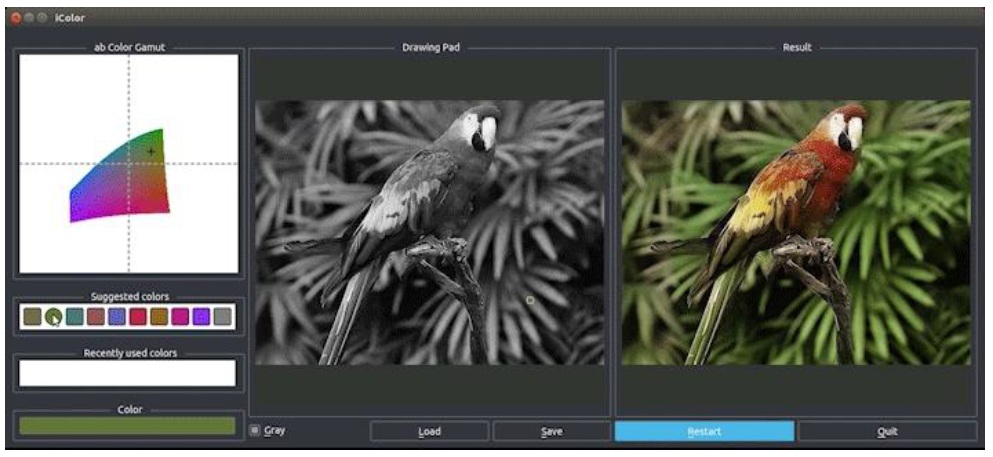Generative Adversarial Networks (GAN) is a framework for estimating generative models via an adversarial process by training two models simultaneously. A generative model G that captures the data distribution, and a discriminative model D that estimates the probability that a sample came from the training data rather than G. It was proposed and presented in Advances in Neural Information Processing Systems (NIPS) 2014.

# Introduction

• Taxonomy of Machine Learning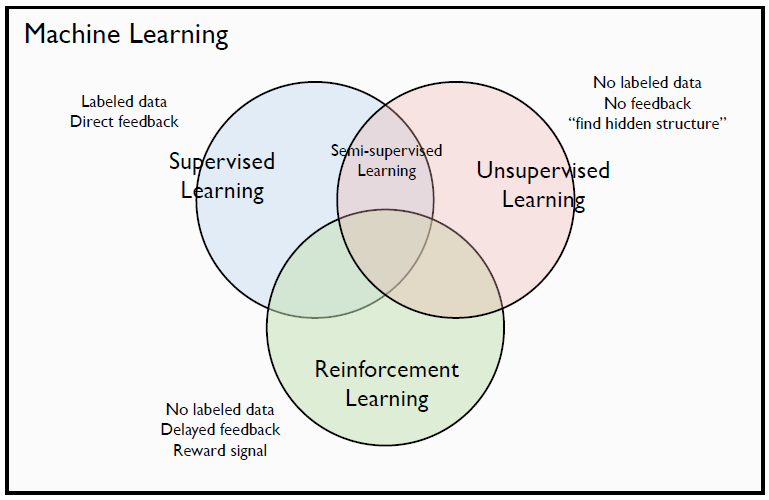• Supervised learning: The discriminative model learns how to classify input to its class.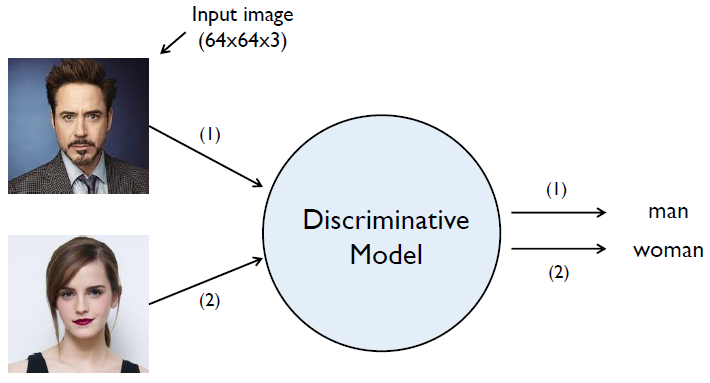• Unsupervised learning: The generative model learns the distribution of training data.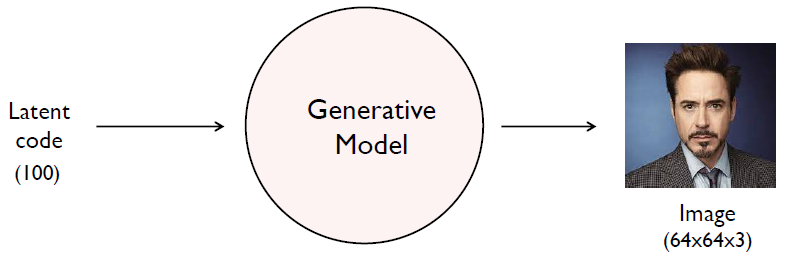• More challenging than supervised learning because there is no label or curriculum which leads self learning
• NN solutions: Boltzmann machine, Auto-encoder, Variation Inference, GAN
• The goal of the generative model is to find a $p_{model}(x)$ that approximates $p_{data}(x)$ well.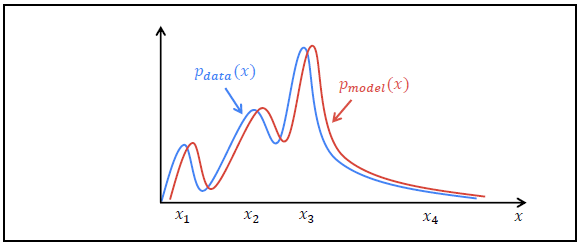## Intuition of GAN

• The discriminator D should classify a real image as real ($D(x)$ close to 1) and a fake image as fake ($D(G(z))$ close to 0).
• The generator G should create an image that is indistinguishable from real to deceive the discriminator ($D(G(z))$ close to 1).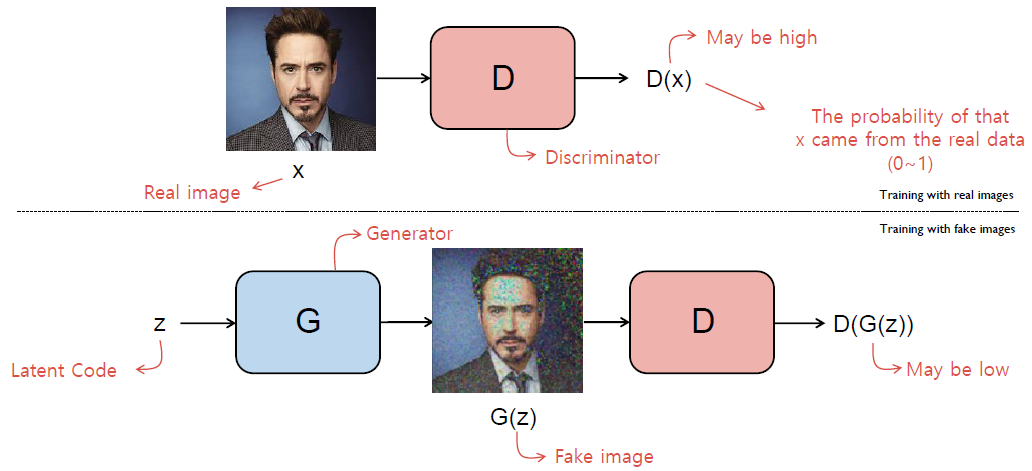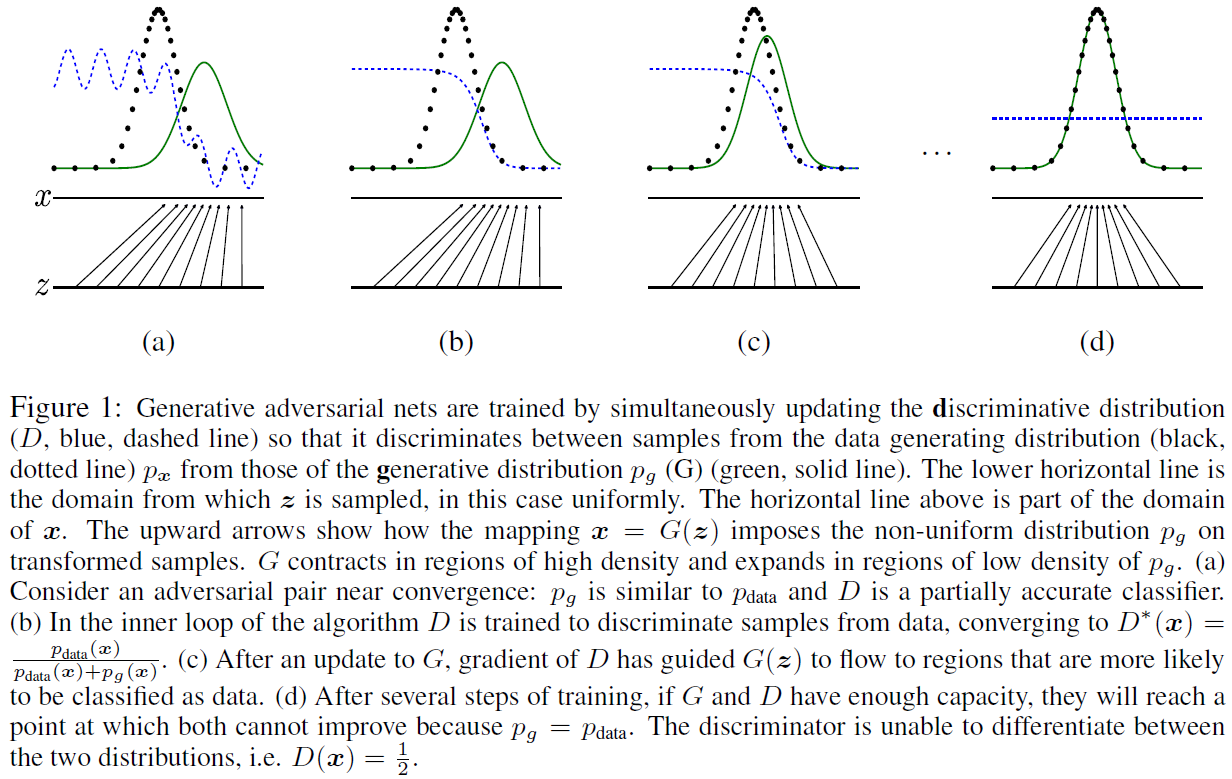## Objective Function

• Objective function of GAN is minimax game of two-player G and D.
• For the discriminator D should maximize $V(D,G)$
• Sample $x$ from real data distribution for $p_{data}(x)$
• Sample latent code $z$ from Gaussian distribution for $p_z(z)$
• $V(D,G)$ is maximum when $D(x)=1$ and $D(G(z))=0$
• For the generator G should minimize $V(D,G)$
• G is independent of $\mathbb{E}_{x\sim p_{data}~(x)}[log D(x)]$
• $V(D,G)$ is minimum when $D(G(z))=1$
• Saturating problem
• In practice, early in learning, when G is poor, D can reject samples with high confidence because they are clearly different from the training data.
• In this case, the gradient is relatively small at $D(G(z))=0$ which makes $\log (1-D(G(z)))$ saturates.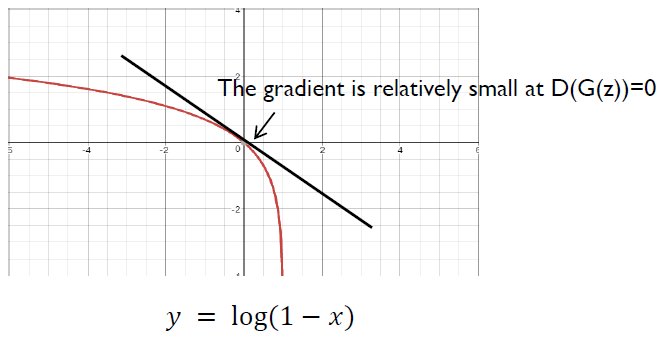• Rather than training G to minimize $\log (1-D(G(z)))$, we can train G to maximize $\log D(G(z))$.
• This objective function results in the same fixed point of the dynamics of G and D but provides much stronger gradients early in learning.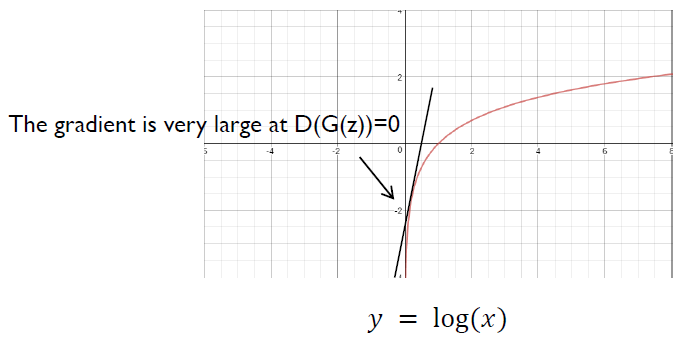• Why does GANs work?
• Because it actually minimizes the distance between the real data distribution $p_{data}$ and the model distribution $p_g$.
• Jensen-Shannon divergence (JSD) is a method of measuring the similarity between two probability distributions based on the Kullback-Leibler divergence (KL).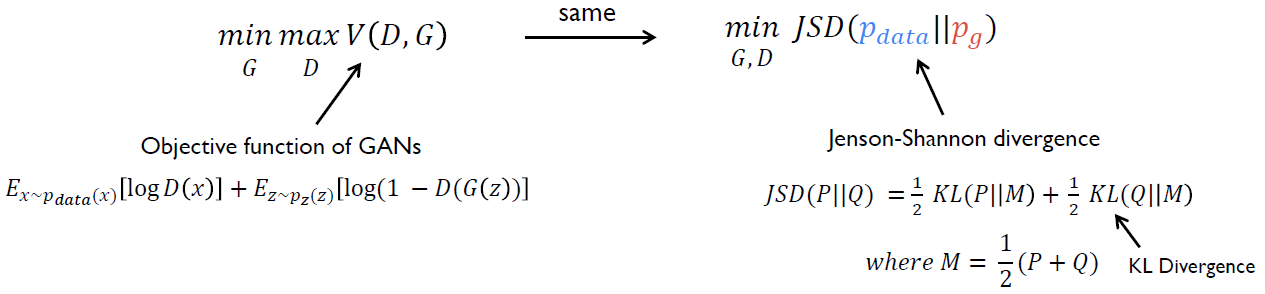# Variants of GAN

## Deep Convolutional GAN (DCGAN), 2015

• DCGAN used convolution for discriminator and deconvolution for generator.
• It is stable to train in most settings compared to GANs.
• DCGAN used the trained discriminators for image classification tasks, showing competitive performance with other unsupervised algorithms.
• Specific filters of DCGAN have learned to draw specific objects.
• The generators have interesting vector arithmetic properties allowing for easy manipulation of many semantic qualities of generated samples.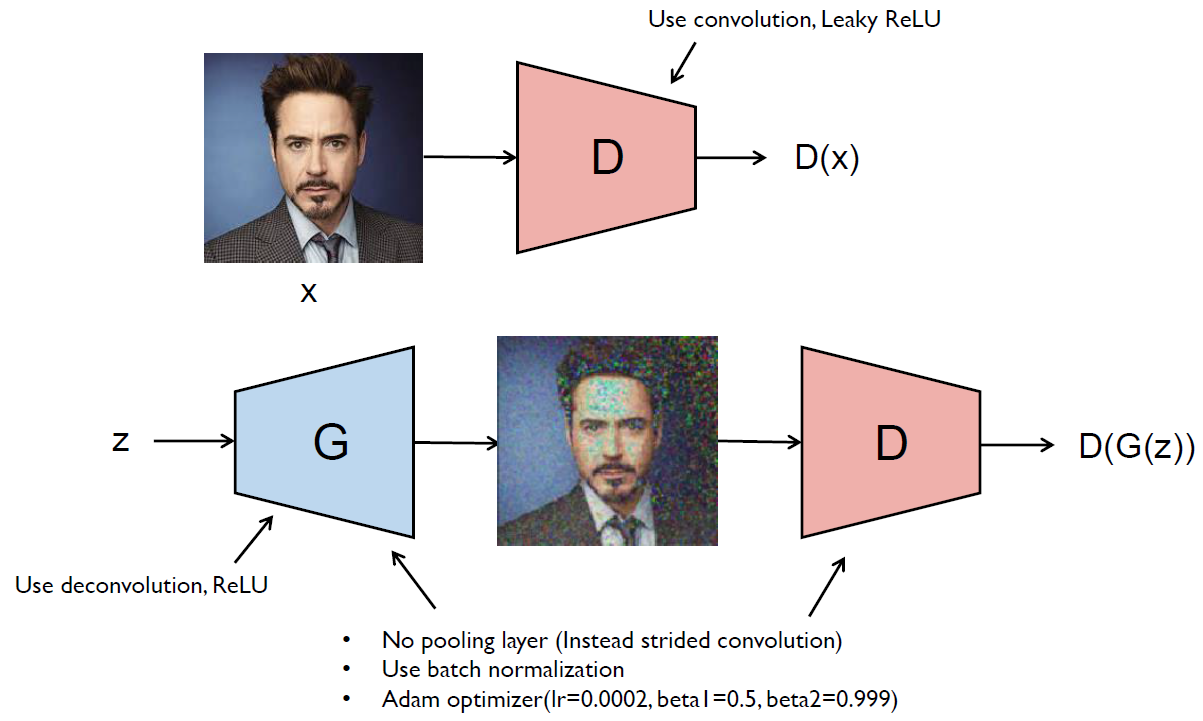• Latent vector arithmetic
• They showed consistent and stable generations that semantically obeyed the linear arithmetic including object manipulation and face pose.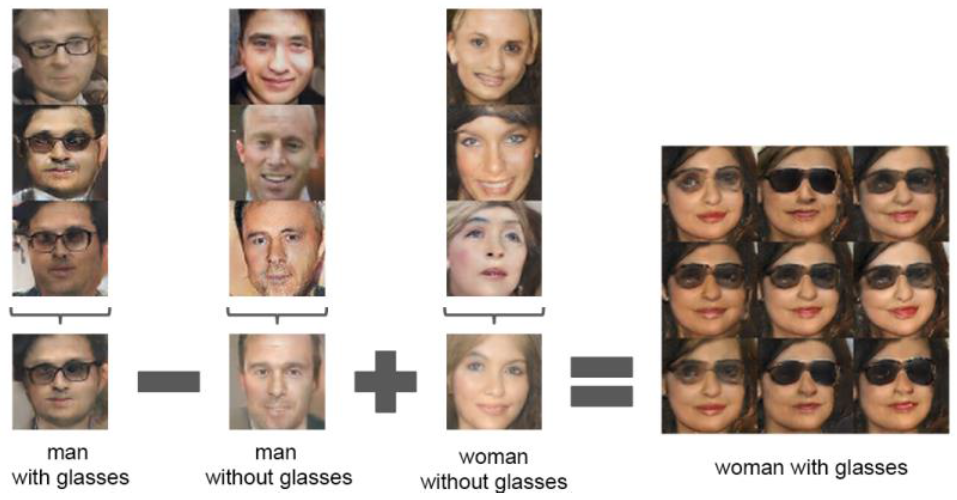## Least Squares GAN (LSGAN), 2016

• LSGAN adopt the least squares loss function for the discriminator instead of cross entropy loss function of GAN.
• Since, cross entropy loss function may lead to the vanishing gradient problem.
• LSGANs are able to generate higher quality images than regular GANs.
• LSGANs performs more stable during the learning process.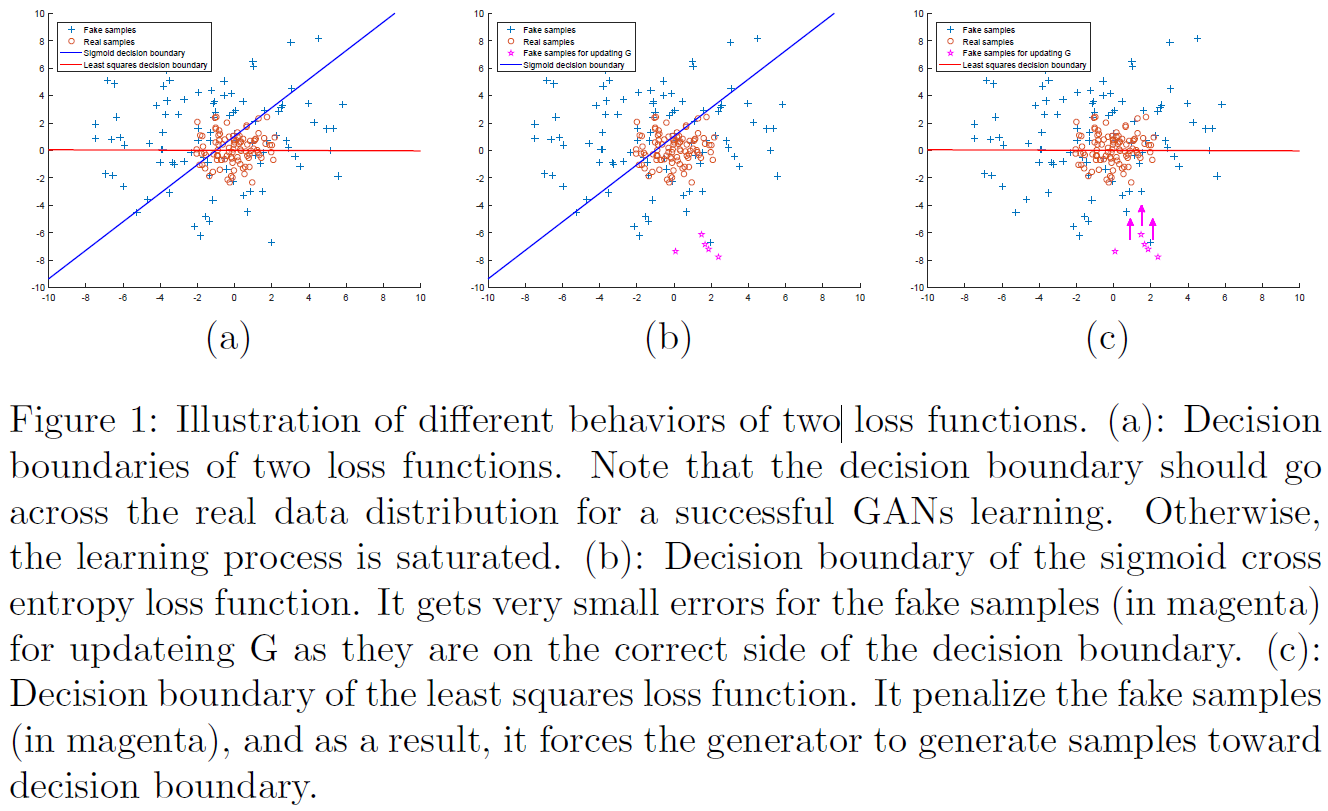## Semi-Supervised GAN (SGAN), 2016

• SGAN extend GANs that allows them to learn a generative model and a classifier simultaneously.
• SGAN improves classification performance on restricted data sets over a baseline classifier with no generative component.
• SGAN can significantly improve the quality of the generated samples and reduce training times for the generator.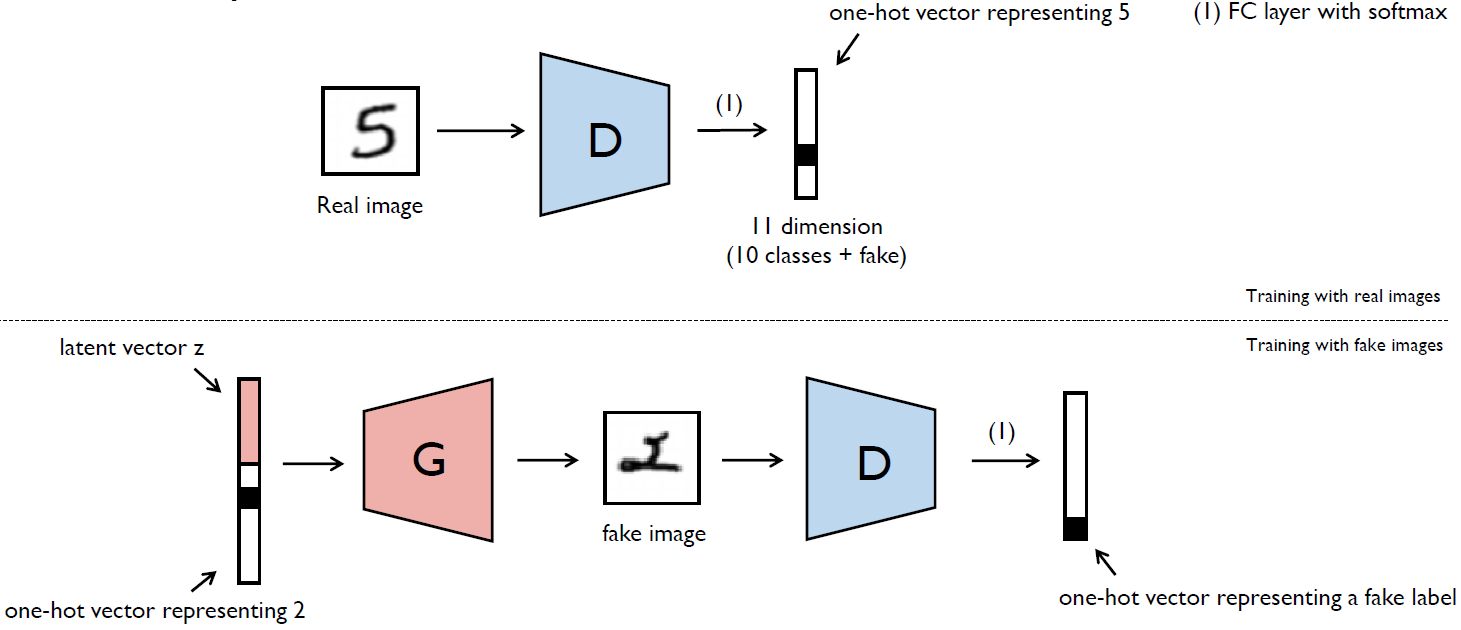## Auxiliary Classifier GAN (ACGAN), 2016

• ACGAN is added more structure to the GAN latent space along with a specialized cost function results in higher quality samples.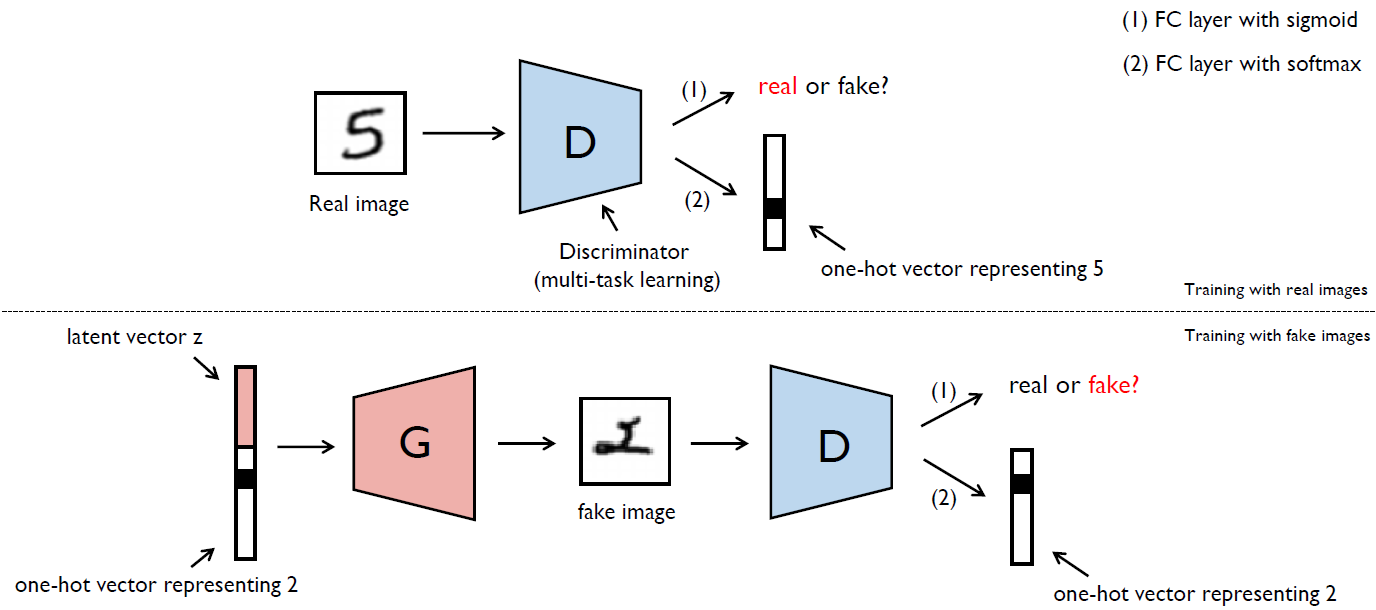# Extensions of GAN

## CycleGAN: Unpaired Image-to-Image Translation

• CycleGAN presents a GAN model that transfer an image from a source domain A to a target domain B in the absence of paired examples.
• The generator $G_{AB}$ should generates a horse from the zebra to deceive the discriminator $D_B$.
• $G_{BA}$ generates a reconstructed image of domain A which makes the shape to be maintained when $G_{AB}$ generates a horse image from the zebra.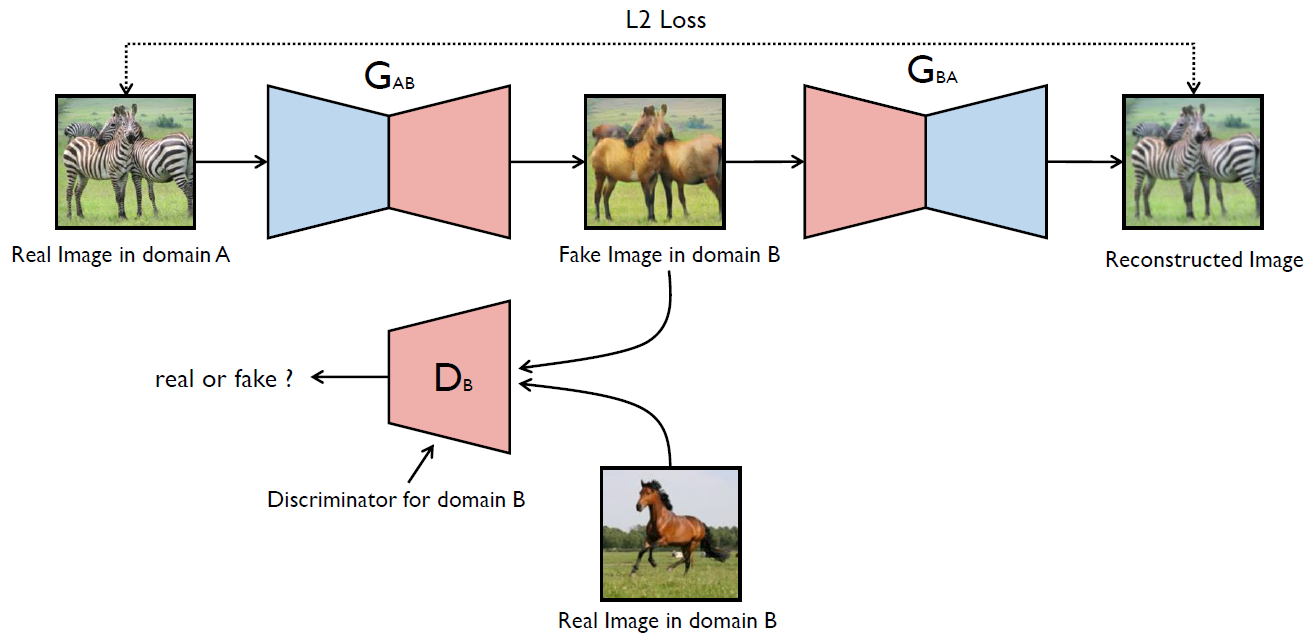• Result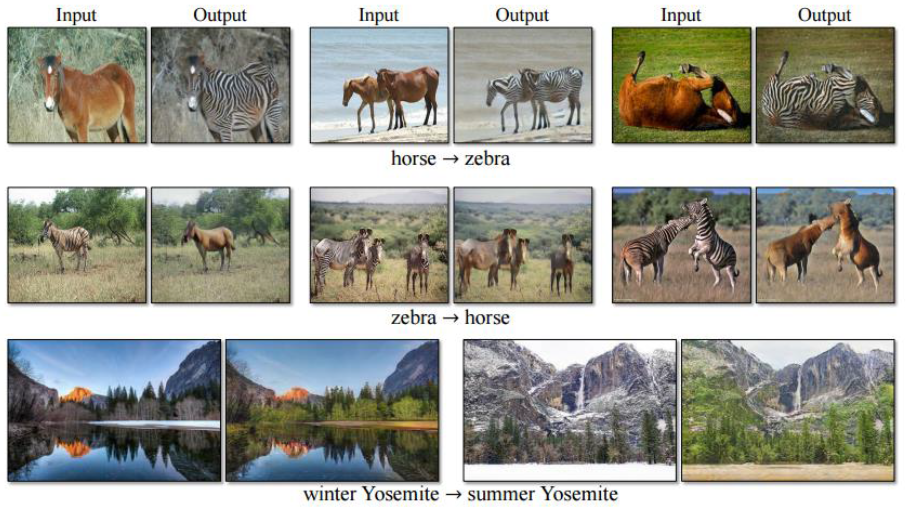## StackGAN: Text to Photo-realistic Image Synthesis

• StackGAN generate $256 \times 256$ photo-realistic images conditioned on text descriptions.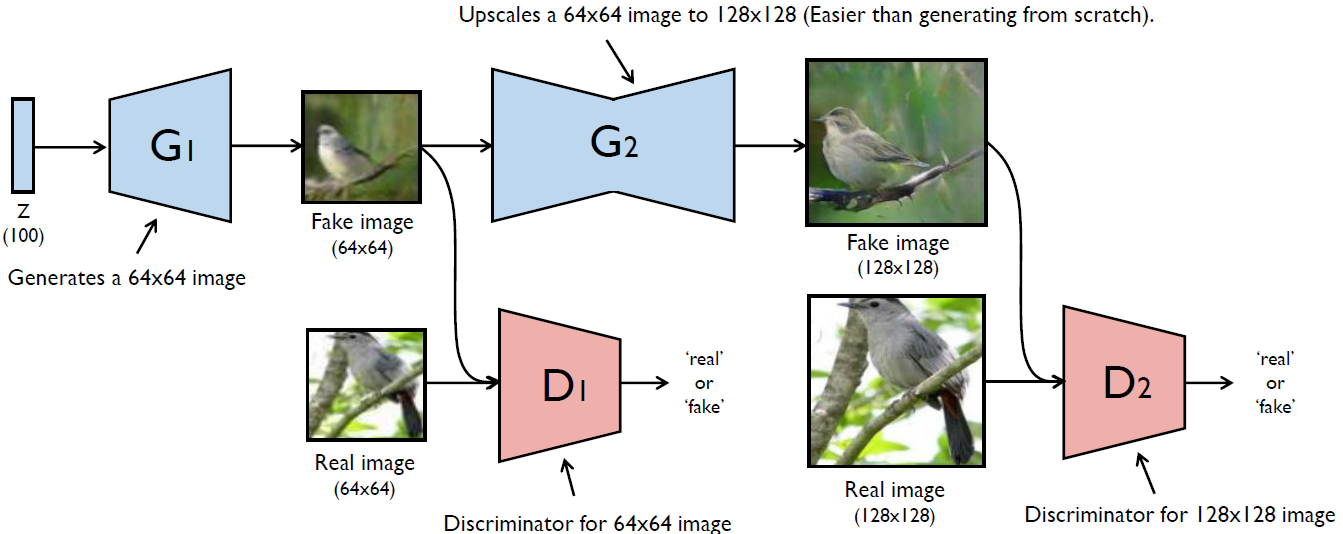• Result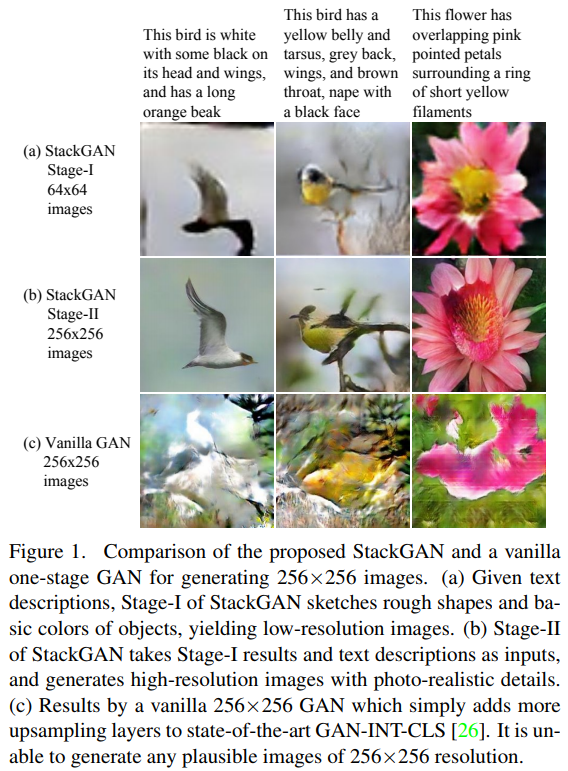## Latest work

• Visual Attribute Transfer
• Jing Liao et al. Visual Attribute Transfer through Deep Image Analogy, 2017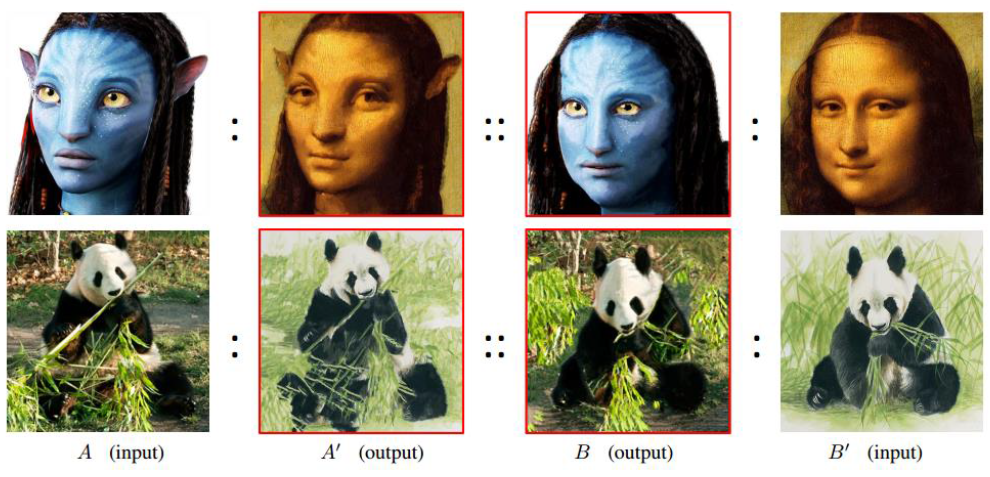• User-Interactive Image Colorization
• Richard Zhang et al. Real-Time User-Guided Image Colorization with Learned Deep Prioirs, 2017